Representations of Surfaces (part 1):  z=f(x,y)

Surface of the form: (z=f(x,y))

We construction an example function of the form z = U(S,V) for display with different kinds of superimposed information.

In:=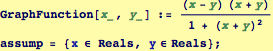A default view of the surface.

In:=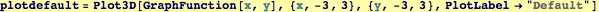Out=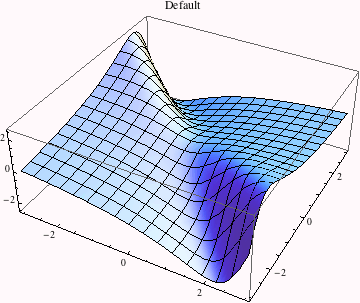Plotting the surface with levels of constant height superimposed and colored according to its height.

In:=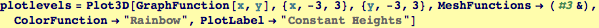Out=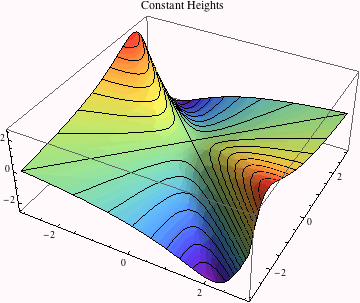This function will be used to paint surface according to an angular variable.

In:=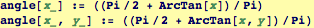Drawing circles on the plot and coloring accoding to the angle; this is superposing a visualization of the cylindrical coordinate system.

In:=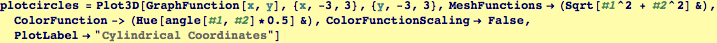Out=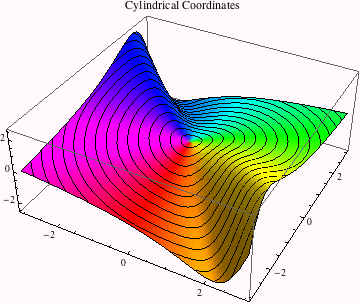Here we write a function to calculate the curvature of f(x,y) as a function of x and y. We will use this to draw lines of constant curvature.

In:=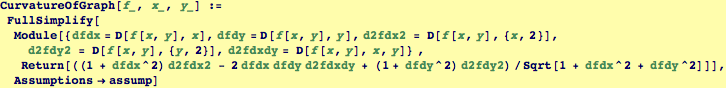This is a moderately advanced application---here I define a function symbolically by evaluating the curvature function, this will be passed to Plot3D as the MeshFunction.

In:=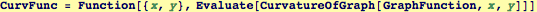Out=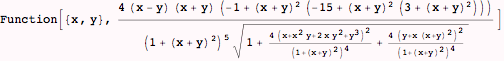Created by Wolfram Mathematica 6.0  (28 September 2007)